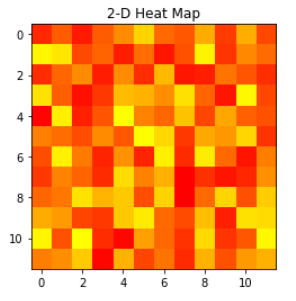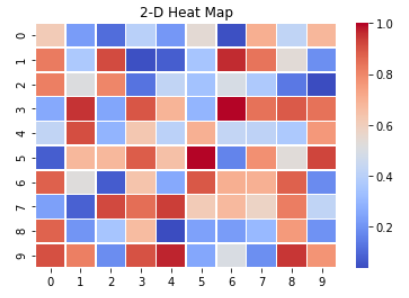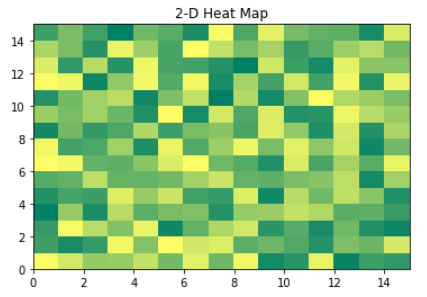Open in App
Not now

# How to draw 2D Heatmap using Matplotlib in python?

• Last Updated : 26 Nov, 2020

A 2-D Heatmap is a data visualization tool that helps to represent the magnitude of the phenomenon in form of colors. In python, we can plot 2-D Heatmaps using Matplotlib package. There are different methods to plot 2-D Heatmaps, some of them are discussed below.

Method 1: Using matplotlib.pyplot.imshow() Function

Syntax: matplotlib.pyplot.imshow(X, cmap=None, norm=None, aspect=None, interpolation=None, alpha=None, vmin=None,
vmax=None, origin=None, extent=None, shape=<deprecated parameter>, filternorm=1, filterrad=4.0,
imlim=<deprecated parameter>, resample=None, url=None, \*, data=None, \*\*kwargs)

## Python3

 `# Program to plot 2-D Heat map ` `# using matplotlib.pyplot.imshow() method ` `import` `numpy as np ` `import` `matplotlib.pyplot as plt ` ` `  `data ``=` `np.random.random(( ``12` `, ``12` `)) ` `plt.imshow( data , cmap ``=` `'autumn'` `, interpolation ``=` `'nearest'` `) ` ` `  `plt.title( ``"2-D Heat Map"` `) ` `plt.show() `

Output:Method 2: Using Seaborn Library

For this we use seaborn.heatmap() function

Syntax: seaborn.heatmap(data, *, vmin=None, vmax=None, cmap=None, center=None, robust=False,annot=None,
fmt=’.2g’, annot_kws=None, linewidths=0, linecolor=’white’, cbar=True, cbar_kws=None, cbar_ax=None,
square=False, xticklabels=’auto’, yticklabels=’auto’, mask=None, ax=None, **kwargs)

## Python3

 `# Program to plot 2-D Heat map ` `# using seaborn.heatmap() method ` `import` `numpy as np ` `import` `seaborn as sns ` `import` `matplotlib.pylab as plt ` ` `  `data_set ``=` `np.random.rand( ``10` `, ``10` `) ` `ax ``=` `sns.heatmap( data_set , linewidth ``=` `0.5` `, cmap ``=` `'coolwarm'` `) ` ` `  `plt.title( ``"2-D Heat Map"` `) ` `plt.show() `

Output:Method 3: Using matplotlib.pyplot.pcolormesh() Function

Syntax: matplotlib.pyplot.pcolormesh(*args, alpha=None, norm=None, cmap=None, vmin=None, vmax=None,

## Python3

 `# Program to plot 2-D Heat map ` `# using matplotlib.pyplot.pcolormesh() method ` `import` `matplotlib.pyplot as plt ` `import` `numpy as np ` ` `  `Z ``=` `np.random.rand( ``15` `, ``15` `) ` ` `  `plt.pcolormesh( Z , cmap ``=` `'summer'` `) ` ` `  `plt.title( ``'2-D Heat Map'` `) ` `plt.show() `

Output:My Personal Notes arrow_drop_up
Related Articles